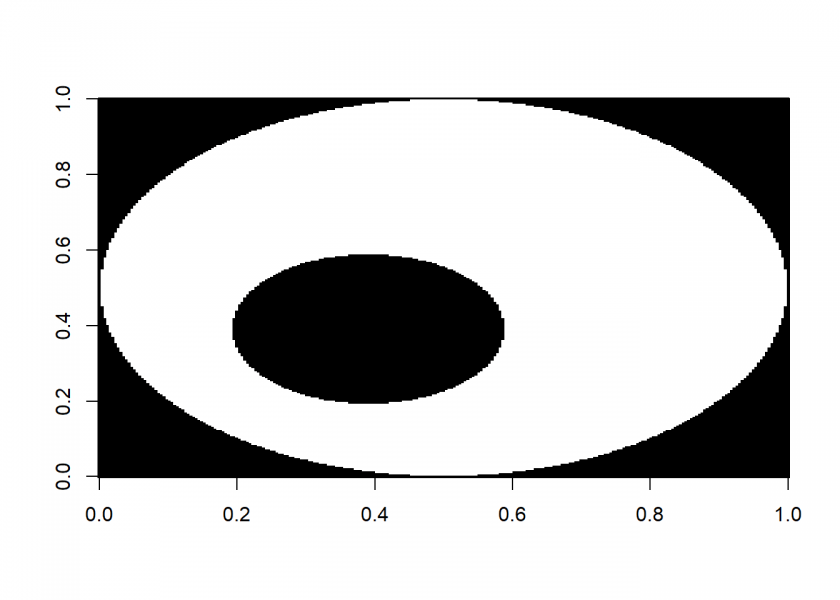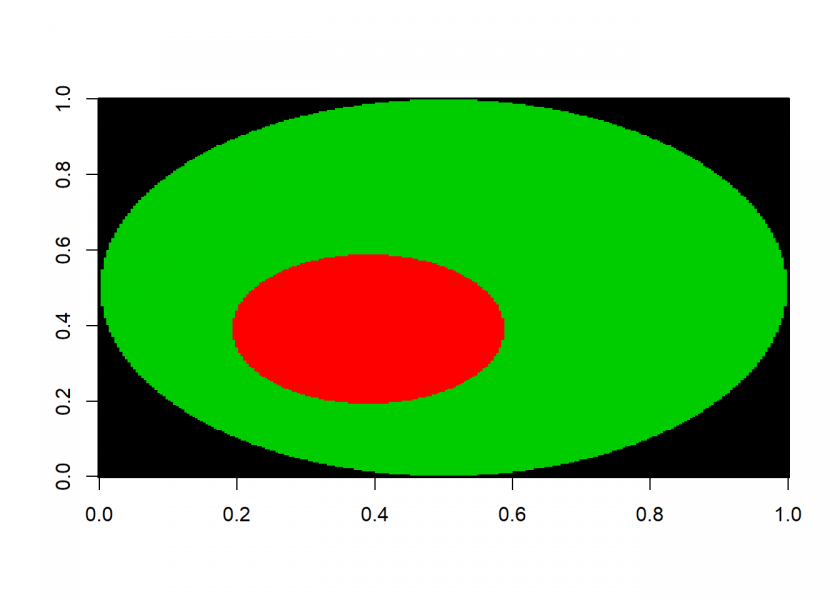Want to share your content on R-bloggers? click here if you have a blog, or here if you don't.A few months ago I published a post on recursion: To understand Recursion you have to understand Recursion…. In this post we will see how to use recursion to fill free areas of an image with colour, the caveats of recursion and how to transform a recursive algorithm into a loop-based version using a queue – so read on…

The recursive version of the painting algorithm we want to examine here is very easy to understand, Wikipedia gives the pseudocode of the so called flood-fill algorithm:

Flood-fill (node, target-color, replacement-color):

• If target-color is equal to replacement-color, return.
• If the color of node is not equal to target-color, return.
• Set the color of node to replacement-color.
• Perform Flood-fill (one step to the south of node, target-color, replacement-color).
Perform Flood-fill (one step to the north of node, target-color, replacement-color).
Perform Flood-fill (one step to the west of node, target-color, replacement-color).
Perform Flood-fill (one step to the east of node, target-color, replacement-color).
• Return.

The translation into R couldn’t be any easier:

```floodfill <- function(row, col, tcol, rcol) {
if (tcol == rcol) return()
if (M[row, col] != tcol) return()
M[row, col] <<- rcol
floodfill(row - 1, col    , tcol, rcol) # south
floodfill(row + 1, col    , tcol, rcol) # north
floodfill(row    , col - 1, tcol, rcol) # west
floodfill(row    , col + 1, tcol, rcol) # east
return("filling completed")
}
```

We take the image from Wikipedia as an example:

```M <- matrix(c(1, 1, 1, 1, 1, 1, 1, 1, 1,
1, 0, 0, 0, 1, 0, 0, 0, 1,
1, 0, 0, 0, 1, 0, 0, 0, 1,
1, 0, 0, 1, 0, 0, 0, 0, 1,
1, 1, 1, 0, 0, 0, 1, 1, 1,
1, 0, 0, 0, 0, 1, 0, 0, 1,
1, 0, 0, 0, 1, 0, 0, 0, 1,
1, 0, 0, 0, 1, 0, 0, 0, 1,
1, 1, 1, 1, 1, 1, 1, 1, 1), 9, 9)
image(M, col = c(0, 1))
```We now fill the three areas with three different colours and then plot the image again:

```startrow <- 5; startcol <- 5
floodfill(startrow, startcol, 0, 2)
##  "filling completed"

startrow <- 3; startcol <- 3
floodfill(startrow, startcol, 0, 3)
##  "filling completed"

startrow <- 7; startcol <- 7
floodfill(startrow, startcol, 0, 4)
##  "filling completed"

image(M, col = 1:4)
```This seems to work pretty well but the problem is that the more nested the algorithm becomes the bigger the stack has to be – which could lead to overflow errors. One comment on my original post on recursion read:

just keep in mind that recursion is useful in industrial work only if tail optimization is supported. otherwise your code will explode at some indeterminate time in the future. […]

One possibility is to increase the size of the stack with `options(expressions = 10000)` but even this may not be enough. Therefore we transform our recursive algorithm into a loop-based one and use a queue instead of a stack! The pseudocode from Wikipedia:

Flood-fill (node, target-color, replacement-color):

• If target-color is equal to replacement-color, return.
• If color of node is not equal to target-color, return.
• Set the color of node to replacement-color.
• Set Q to the empty queue.
• Add node to the end of Q.
• While Q is not empty:
• Set n equal to the first element of Q.
• Remove first element from Q.
• If the color of the node to the west of n is target-color,
set the color of that node to replacement-color and add that node to the end of Q.
• If the color of the node to the east of n is target-color,
set the color of that node to replacement-color and add that node to the end of Q.
• If the color of the node to the north of n is target-color,
set the color of that node to replacement-color and add that node to the end of Q.
• If the color of the node to the south of n is target-color,
set the color of that node to replacement-color and add that node to the end of Q.
• Continue looping until Q is exhausted.
• Return.

Because of the way the algorithm fills areas it is also called forest fire. Again, the translation into valid R code is straightforward:

```floodfill <- function(row, col, tcol, rcol) {
if (tcol == rcol) return()
if (M[row, col] != tcol) return()
Q <- matrix(c(row, col), 1, 2)
while (dim(Q) > 0) {
n <- Q[1, , drop = FALSE]
west  <- cbind(n    , n - 1)
east  <- cbind(n    , n + 1)
north <- cbind(n + 1, n    )
south <- cbind(n - 1, n    )
Q <- Q[-1, , drop = FALSE]
if (M[n] == tcol) {
M[n] <<- rcol
if (M[west] == tcol)  Q <- rbind(Q, west)
if (M[east] == tcol)  Q <- rbind(Q, east)
if (M[north] == tcol) Q <- rbind(Q, north)
if (M[south] == tcol) Q <- rbind(Q, south)
}
}
return("filling completed")
}
```

As an example we will use a much bigger picture (it can be downloaded from here: Unfilledcirc.png):

```library(png)
M <- img[ , , 1]
M <- ifelse(M < 0.5, 0, 1)
M <- rbind(M, 0)
M <- cbind(M, 0)
image(M, col = c(1, 0))
```And now for the filling:

```startrow <- 100; startcol <- 100
floodfill(startrow, startcol, 0, 2)
##  "filling completed"

startrow <- 50; startcol <- 50
floodfill(startrow, startcol, 1, 3)
##  "filling completed"

image(M, col = c(1, 0, 2, 3))
```As you can see, with this version of the algorithm much bigger areas can be filled!

I also added both R implementations to the respective section of Rosetta Code: Bitmap/Flood fill.